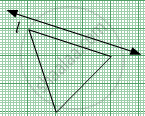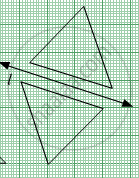# Along the Figure Shown Below, a Line L Has Been Drawn. Complete the Symmetrical Figure by Drawing a Figure on the Other Side Such that the Line L Becomes the Line of Symmetry. - Mathematics

Sum

Along the figure shown below, a line l has been drawn. Complete the symmetrical figure by drawing a figure on the other side such that the line l becomes the line of symmetry.#### SolutionConcept: Drawing a Symmetric Figure on a Graph
Is there an error in this question or solution?

#### APPEARS IN

Balbharati Mathematics 6th Standard Maharashtra State Board
Chapter 7 Symmetry
Practice Set 21 | Q 1.3 | Page 21

Share